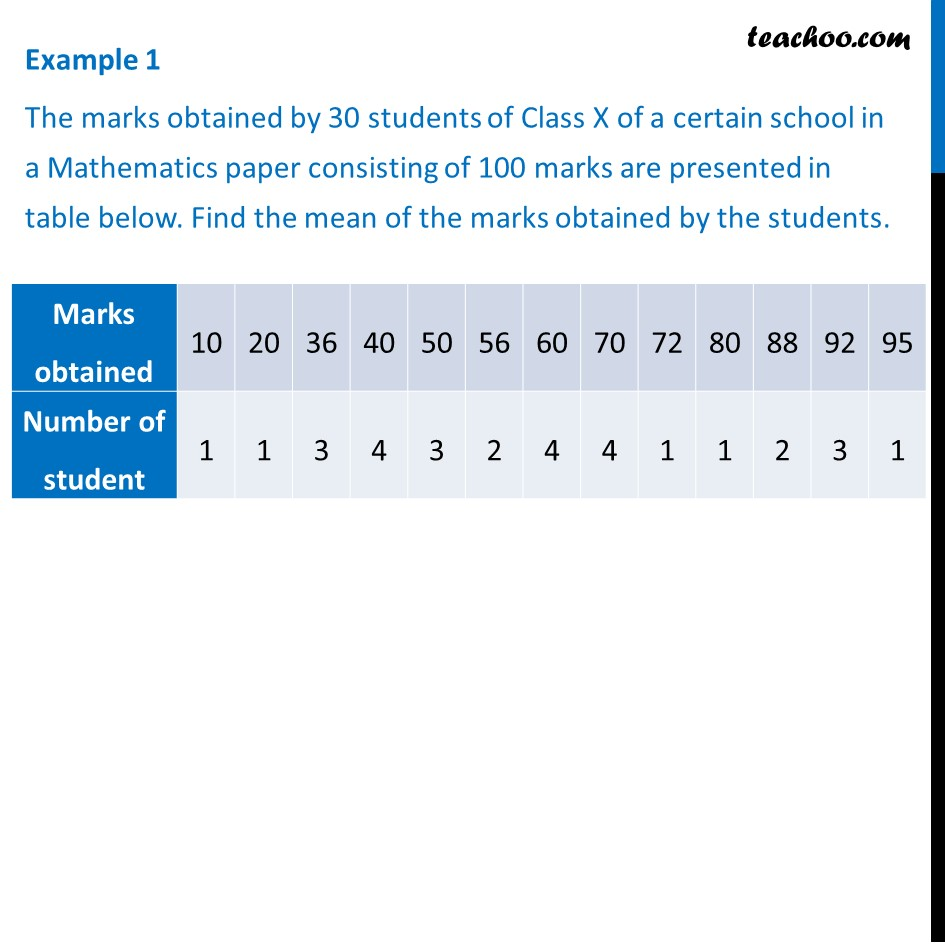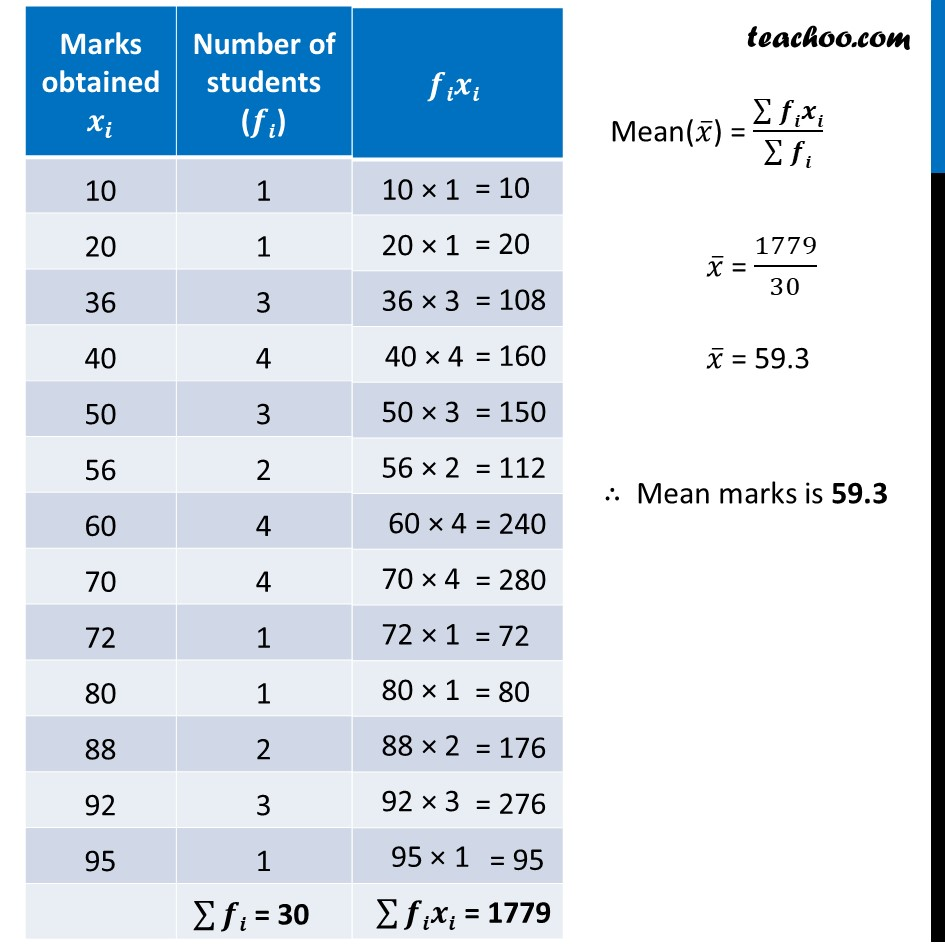Examples

Chapter 14 Class 10 Statistics
Serial order wiseGet live Maths 1-on-1 Classs - Class 6 to 12

### Transcript

Example 1 The marks obtained by 30 students of Class X of a certain school in a Mathematics paper consisting of 100 marks are presented in table below. Find the mean of the marks obtained by the students. Mean(𝑥 ̅) = (∑▒𝒇𝒊𝒙𝒊)/(∑▒𝒇𝒊) 𝑥 ̅ = 1779/30 𝑥 ̅ = 59.3 ∴ Mean marks is 59.3